# Bread

5 of the same bread has the same weight as three bread and 4 kg of fruit. What weight has one bread?

Result

x =  2 kg

#### Solution:

5x = 3x + 4

2x = 4

x = 2

Calculated by our simple equation calculator.

Leave us a comment of example and its solution (i.e. if it is still somewhat unclear...):

Showing 0 comments:Be the first to comment!#### To solve this example are needed these knowledge from mathematics:

Do you have a linear equation or system of equations and looking for its solution? Or do you have quadratic equation?

## Next similar examples:

1. NumberI think the number. If I add to its third seven I get same as when to its quarter add 8. Which is the number?
2. Brick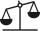Isosceles scale has on one side all brick and second weight 1 kg and 1/4 of brick. The balance is in equilibrium. What is the weight of a brick?
3. Sumo competition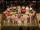In the competition for the heaviest man was 5 competitors. The first three together weighed 553 kg. What is the minimal weigt of winner, if a contestant weighed with an accuracy of one kilogram.
4. Dropped sheetsThree consecutive sheets dropped from the book. The sum of the numbers on the pages of the dropped sheets is 273. What number has the last page of the dropped sheets?
5. Coal storage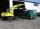The coal storage distribute received coal shipment within three days. The first day distribute third of the shipments, the second day of two-fifths of the rest and the third day 300 tons of coal. How many tons of coal distributed first and second day?
6. NormThree workers planted 3555 seedlings of tomatoes in one dey. First worked at the standard norm, the second planted 120 seedlings more and the third 135 seedlings more than the first worker. How many seedlings were standard norm?
7. Unknown number 11That number increased by three equals three times itself?
8. Can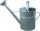Watering can full of water weighs 10 kg. Half-full can weighs 5.5 kg. How much weigh can?
9. Two bricks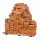One brick weighs 1kg and half a brick, how many weigh two bricks?
10. Negative in equation2x + 3 + 7x = – 24, what is the value of x?
11. FlourKim needs 3/4 cup of flour to make 12 cookies. How much flour would she need to make 60 cookies?
12. Find xSolve: if 2(x-1)=14, then x= (solve an equation with one unknown)
13. Simple equationSolve the following simple equation: 2. (4x + 3) = 2-5. (1-x)
14. Forest nurseryIn the forest nursery after winter, they found that 1/10 stems died out of them. For them, they land 193 new spruces. How many spruces are in the forest nursery?
15. Simple equationSolve for x: 3(x + 2) = x - 18
16. Equation 29Solve next equation: 2 ( 2x + 3 ) = 8 ( 1 - x) -5 ( x -2 )
17. CakesGrandmother baked cakes. Half of its was poppy, quarter with plum jam and 16 cheesecakes. How many cakes she baked in total?